# TABLES 1 TO 20Multiplication is possible only on the basis of tables at least of numbers 1 to 20. Tables are not just maths but read in English. For instance, (2x1=2) is read as (2 ones are 2). So tables are beyond maths. You take any number table; it should be multiplied with other numbers to get a resultant number. There is enormous calculation happening within you to understand it but medium effort has to be put to learn tables 1 to 20 at least.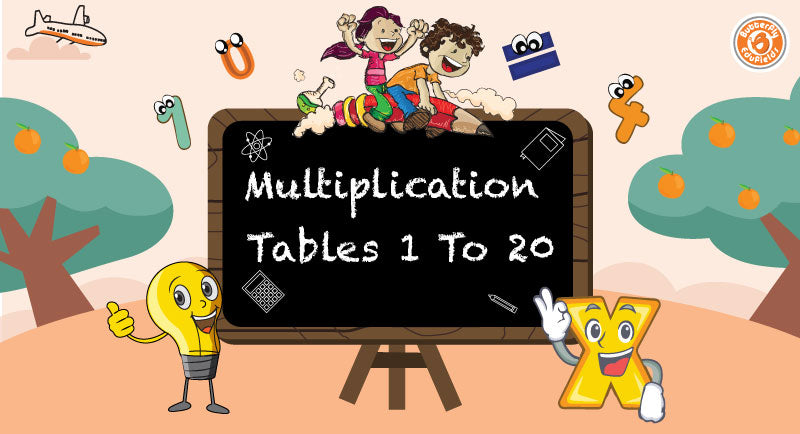### 20+ Science Experiments Kit for Kids, Jumbo STEM DIY Educational Electronic Building Construction Activity Toys for Boys Girls Age 5+ | Motor Machines

Many use calculators for multiplication functions which doesn’t test their ability to think. So we better learn tables with at least 20 numbers to ease the process.

Few other learnings with the same number multiplied by itself one time gives a square. Twice by itself gives a cube and thereon to the power of infinity. (2x2=2^2 =4) and (3x3x3=3^3=27) …. etc. Even the prime, Even, Odd number concepts are completely dependent on multiplication tables. Now that’s the essentiality of learning tables.

Learning tables are similar to memory games. We try to memorize numbers. During childhood, when we do it we make it a play. But the significance of it appears everywhere in the career. It helps in improving speed during competitive exams or day to day operations. So let’s brush up our multiplication table knowledge. On a scale of 1 to 10, where are you standing in terms of knowing tables? If there are less than 5 no worries, let us become experts. Let’s learn a few insights about each table.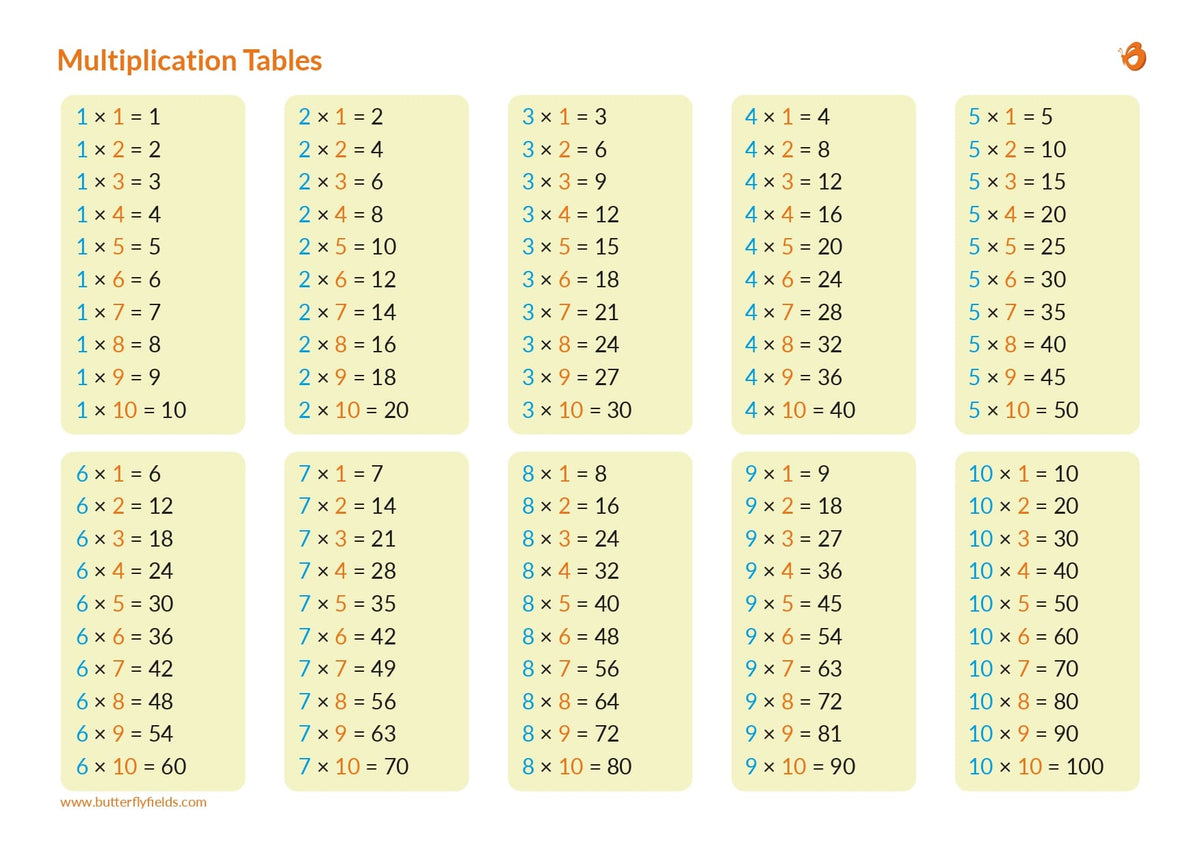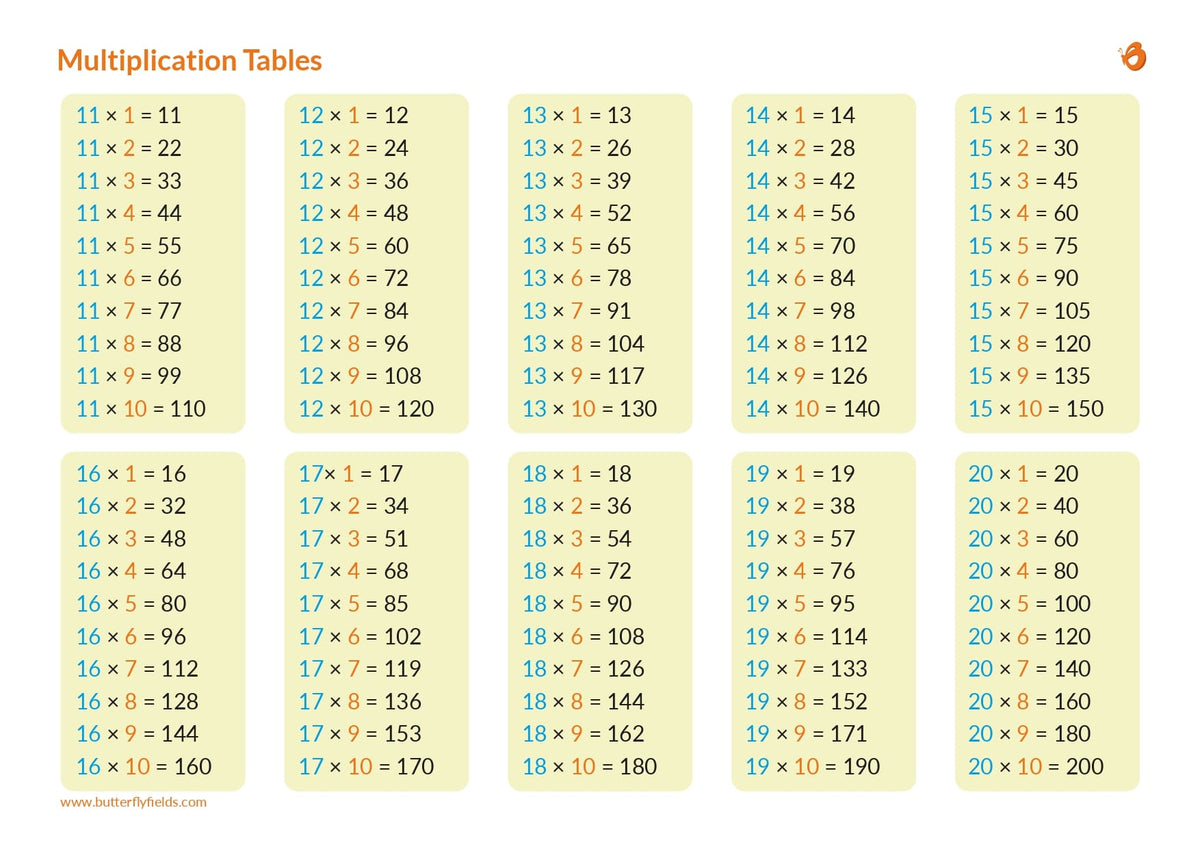## Table of 1 to 5

Table of 1 Table of 2 Table of 3 Table of 4 Table of 5
1 × 1 = 1 2 × 1 = 2 3 × 1 = 3 4 × 1 = 4 5 × 1 = 5
2 × 1 = 2 2 × 2 = 4 3 × 2 = 6 4 × 2 = 8 5 × 2 = 10
3 × 1 = 3 2 × 3 = 6 3 × 3 = 9 4 × 3 = 12 5 × 3 = 15
4 × 1 = 4 2 × 4 = 8 3 × 4 = 12 4 × 4 = 16 5 × 4 = 20
5 × 1 = 5 2 × 5 = 10 3 × 5 = 15 4 × 5 = 20 5 × 5 = 25
6 × 1 =6 2 × 6 = 12 3 × 6 = 18 4 × 6 = 24 5 × 6 = 30
7 × 1 = 7 2 × 7 = 14 3 × 7 = 21 4 × 7 = 28 5 × 7 = 35
8 × 1 = 8 2 × 8 = 16 3 × 8 = 24 4 × 8 = 32 5 × 8 = 40
9 × 1 = 9 2 × 9 = 18 3 × 9 = 27 4 × 9 = 36 5 × 9 = 45
10 × 1 = 10 2 × 10 = 20 3 × 10 = 30 4 × 10 = 40 5 × 10 = 50

### Multiplication Table 1:

Anything and everything multiplied to 1, becomes that multiplied number. Starting from 0 to ∞ applied to all. There is no such brainstorming in this table compared to others. It’s as easy as 1x banana = 1 banana or 1x football = 1 football and 1x10=10. That’s it! you can feel now you know 1 table. This is easiest out of tables 1 to 20.

### Multiplication Table 2:

This table is slightly interesting because a number multiplied by 2 will be doubled after multiplication. 5 multiplied by 2 becomes 10 which is double of 5. Difference between any two consecutive multiples of 2 is 2 which are called twin numbers which are specific to 2 table. Multiples of 2 are also called even numbers. Hey! congrats now you are aware of it.

### Multiplication Table 3:

Add a number 3 times to itself then it becomes a multiple of 3. Example 2+2+2 = 6. So 2, 3x is 6 so the table can be written 3x2= 6. This method is applied throughout the table. 3 is a prime number but multiples of 3 may or may not be a prime number.

#### Multiplication Table 4:

Have you heard of leap year? Did you know leap year is multiple of year like 2012, 2016,2020, 2024…etc.? table of 4 is just an addition of a number 4 times to itself. Anything multiplied with 4 becomes an even number because 4 itself is an even number.

##### Multiplication Table 5:

Unit digit of multiple of 5 is either 5 or 0. Every time we multiply a number with 5 add 5 to the result value. Very basic table but yet fun to learn. Multiples of 5 are 5,10,15,20,25,30…etc.

###### Table of 6 to 10
Table of 6 Table of 7 Table of 8 Table of 9 Table of 10
6 × 1 = 6 7 × 1 = 7 8 × 1 = 8 9 × 1 = 9 10 × 1 = 10
6 × 2 = 12 7 × 2 = 14 8 × 2 = 16 9 × 2 = 18 10 × 2 = 20
6 × 3 = 18 7 × 3 = 21 8 × 3 = 24 9 × 3 = 27 10 × 3 = 30
6 × 4 = 24 7 × 4 = 28 8 × 4 = 32 9 × 4 = 36 10 × 4 = 40
6 × 5 = 30 7 × 5 = 35 8 × 5 = 40 9 × 5 = 45 10 × 5 = 50
6 × 6 = 36 7 × 6 = 42 8 × 6 = 48 9 × 6 = 54 10 × 6 = 60
6 × 7 = 42 7 × 7 = 49 8 × 7 = 56 9 × 7 = 63 10 × 7 = 70
6 × 8 = 48 7 × 8 = 56 8 × 8 = 64 9 × 8 = 72 10 × 8 = 80
6 × 9 = 54 7 × 9 = 63 8 × 9 = 72 9 × 9 = 81 10 × 9 = 90
6 × 10 = 60 7 × 10 = 70 8 × 10 = 80 9 × 10 = 90 10 × 10 = 100

### Multiplication Table 6:

Add the number 6, n times to itself becomes the multiple of 6. 6 is as equal as 3. Double the multiples of 3 to get multiples of 6. (3x2=6) (6x2=12). If you can learn 3 table easily, then it’s easy for you to master table 6 as well. Try it. Now you have learnt the technique until table 6.

### Multiplication Table 7:

Add the number 7, n times to itself becomes the multiple of 7. 7 is an odd number and alternative multiples of 7 are even and odd. 7,14,21,28,35…etc. Square of 7 is 49 and the cube of 7 is 343. 7 is considered as a sacred number in numerology. It helps in understanding the tables in a certain template.

### Multiplication Table 8:

Number 8 is a double of 4. By doubling the multiples of 4 we get multiples of 8. It is similar to table 6 in understanding. (Refer to table 6). Again multiples of table 8 are even because 8 I even, so am the multiples. Learn through the table chart given. (8x8=64

### Multiplication Table 9:

Now we are going to learn the biggest single digit i.e., 9. 9 is the triple of 3. So, triple the multiple of 3 to get the multiples of 9. E.g. (3x2=6), (9x2=18). So easy to learn isn’t it?

### Multiplication Table 10:

Perhaps easiest two-digit table. Table 10 is similar to table 1 but adds a zero to the resultant multiple. E.g. if 1x5=5 then 10x5=50. Or keep on adding 10 to the previous multiple to get the new one. And Finally the maths champ learnt 10 tables.

Let’s look at another 10 tables in the process of learning tables 1 to 20

### Table of 11 to 15

Table of 11 Table of 12 Table of 13 Table of 14Table of 15
11 × 1 = 11 12 × 1 = 12 13 × 1 = 13 14 × 1 = 14 15 × 1 = 15
11 × 2 = 22 12 × 2 = 24 13 × 2 = 26 14 × 2 = 28 15 × 2 = 30
11 × 3 = 33 12 × 3 = 36 13 × 3 = 39 14 × 3 = 42 15 × 3 = 45
11 × 4 = 44 12 × 4 = 48 13 × 4 = 52 14 × 4 = 56 15 × 4 = 60
11 × 5 = 55 12 × 5 = 60 13 × 5 = 65 14 × 5 = 70 15 × 5 = 75
11 × 6 = 66 12 × 6 = 72 13 × 6 = 78 14 × 6 = 84 15 × 6 = 90
11 × 7 = 77 12 × 7 = 84 13 × 7 = 91 14 × 7 = 98 15 × 7 = 105
11 × 8 = 88 12 × 8 = 96 13 × 8 = 104 14 × 8 = 112 15 × 8 = 120
11 × 9 = 99 12 × 9 = 108 13 × 9 = 117 14 × 9 = 126 15 × 9 = 135
11 × 10 = 110 12 × 10 = 120 13 × 10 = 130 14 × 10 = 140 15 × 10 = 150

### Multiplication Table 11:

Number 11 is just 1 beside 1. Sounds funny but not. Add 11 to itself N number of times. You get 11,22,33,44,55…etc. Now all the multiples are the same number written twice. Easy to memorize and dispense when required.

### Multiplication Table 12:

Number 12 is used as a dozen in the general market. But 12 is easily understood when you understand hours. 12 hours, 24 hours…etc. it is referred to as half a day. The Multiples of 12 are 12,24,36,48…etc. Most of the tables in our childhood learnt until x12. So it gives you nostalgia for those days.

### Multiplication Table 13:

13 is a unique number from all other numbers because it’s a prime number and you have never heard of it in any other tables. It Is slightly difficult to remember but you can make it by repeating it every day. Multiples of 13 are 13,26,39,52,65…etc.

### Multiplication Table 14:

14 is multiple of 7 so doubling multiples of 7 gives you multiples of 14. Multiples of 7 are 7, 14,21,28… doubling it we get 14,28,42,56…etc. So rather easy to say starting from 14 every alternative multiple of 7 is a multiple of 14. Maths is easy. Isn’t it?

### Multiplication Table 15:

15 Multiplication Table 15 is extremely important for students in grades 8 and up to learn. When you have 15 tables at your fingertips, tackling large mathematical problems becomes easier and faster, saving you time and increasing your interest in the topic. If you wish to memorise 15 tables like a poem, look at the part below for a shortcut to memorise the tables. If you enjoy Math class and want to be ahead of your peers, memorising a table of 15 would be beneficial.

When you are promoted to 8th Standard, it may appear that learning 15 tables is difficult, but this is a myth; once learned, you will be shocked at how simple it is. It would stay in your heart for the rest of your life if you learned it with sincerity. Rather than skipping the tables, memorise Table 15 from the table below.

### Table 16 to 20

Table of 16 Table of 17 Table of 18 Table of 19 Table of 20
16 × 1 = 16 17 × 1 = 17 18 × 1 = 18 19 × 1 = 19 20 × 1 = 20
16 × 2 = 32 17 × 2 = 34 18 × 2 = 36 19 × 2 = 38 20 × 2 = 40
16 × 3 = 48 17 × 3 = 51 18 × 3 = 54 19 × 3 = 57 20 × 3 = 60
16 × 4 = 64 17 × 4 = 68 18 × 4 = 72 19 × 4 = 76 20 × 4 = 80
16 × 5 = 80 17 × 5 = 85 18 × 5 = 90 19 × 5 = 95 20 × 5 = 100
16 × 6 = 96 17 × 6 = 102 18 × 6 = 108 19 × 6 = 114 20 × 6 = 120
16 × 7 = 112 17 × 7 = 119 18 × 7 = 126 19 × 7 = 133 20 × 7 = 140
16 × 8 = 128 17 × 8 = 136 18 × 8 = 144 19 × 8 = 152 20 × 8 = 160
16 × 9 = 144 17 × 9 = 153 18 × 9 = 162 19 × 9 = 171 20 × 9 = 180
16 × 10 = 160 17 × 10 = 170 18 × 10 = 180 19 × 10 = 190 20 × 10 = 200

### Multiplication Table 16:

The table of 16 is fairly simple to learn; we simply double the table of 8 to get the table of 16. We'll apply the strategy in this article to memorise the sixteen table for the rest of our lives.

The table of 16, on the other hand, is more akin to entering a second level of learning a multiplicative pattern of numbers. Memorizing and applying tables allows you to work on issues from various chapters much more quickly. Division, repeated addition of the same integers, fractions, and decimals all benefit from multiplication.

### Multiplication Table 17:

Do not be concerned if you need to memorise the table of 17, as we have devised a simple method for doing so. The 17 table is very significant for students in secondary and higher secondary schools, and if it is memorised, it may be quite useful in solving hard multiplication, division, and arithmetic problems in a matter of minutes. The 17 table is useful not only for solving multiplication problems, but also for determining square roots, LCM, and HCF.

Many students struggle to memorise the table of 17, but once learned, it speeds up long calculations, and if you memorise the table of 17 on the tips, you may save time. We discussed the multiplication table of 17 and shortcuts in this article for your convenience.

### Multiplication Table 18:

Table 18 is regarded as one of the most difficult Math tables below 20. The 18 table is a representation of the number 18 being added to itself over and over again. The number multiplied to 18 will determine how many times it is added to itself. For instance, multiplying 18 by three equals 18 + 18 + 18 = 54. The figures in the 18 times table are tough to memorise and calculate. The 18th multiplication table is provided here to assist pupils in quickly checking and memorising the values.

The table of 18 is provided below in both tabular and graphic format so that students can download and use it as a flashcard to better learn it. The 18 times table is crucial for pupils because it allows them to perform mental computations more quickly and efficiently.

### Multiplication Table 19:

table of 19 Times "Do you know that there is a Chinese version of Go that is played on a grid of 19 x 19 lines?" Ram questioned Ravi. He then proceeded to ask Joy if he could recite the 19-times table. Joy and Sam recited the multiplication table of 19 together, which includes the multiplication of 19 by various whole numbers. The 19 times table can be found in the diagram below.

Learning the 19-digit multiplication table aids you in addressing problems involving the two basic operations of multiplication and division. Examine the 19-times table provided below and try to memorise it

### Multiplication Table 20:

Learning table 20 is a formality. Just to windup, double the multiples of 10 for table 20. 10 becomes 20 so, 20 is 40, 30 is 60, 40 is 80 and so on. Multiples of 20 are 20,40,60,80…etc.

### Conclusion:

After learning the techniques, now you have gained expertise on tables 1 to 20. If we apply these tables 1 to 20 into our fundamental mathematics it's easy and fun to solve more and more problems. It helps in preparing for higher level competitive exams as well.

### FAQ's On Tables 1 to 20: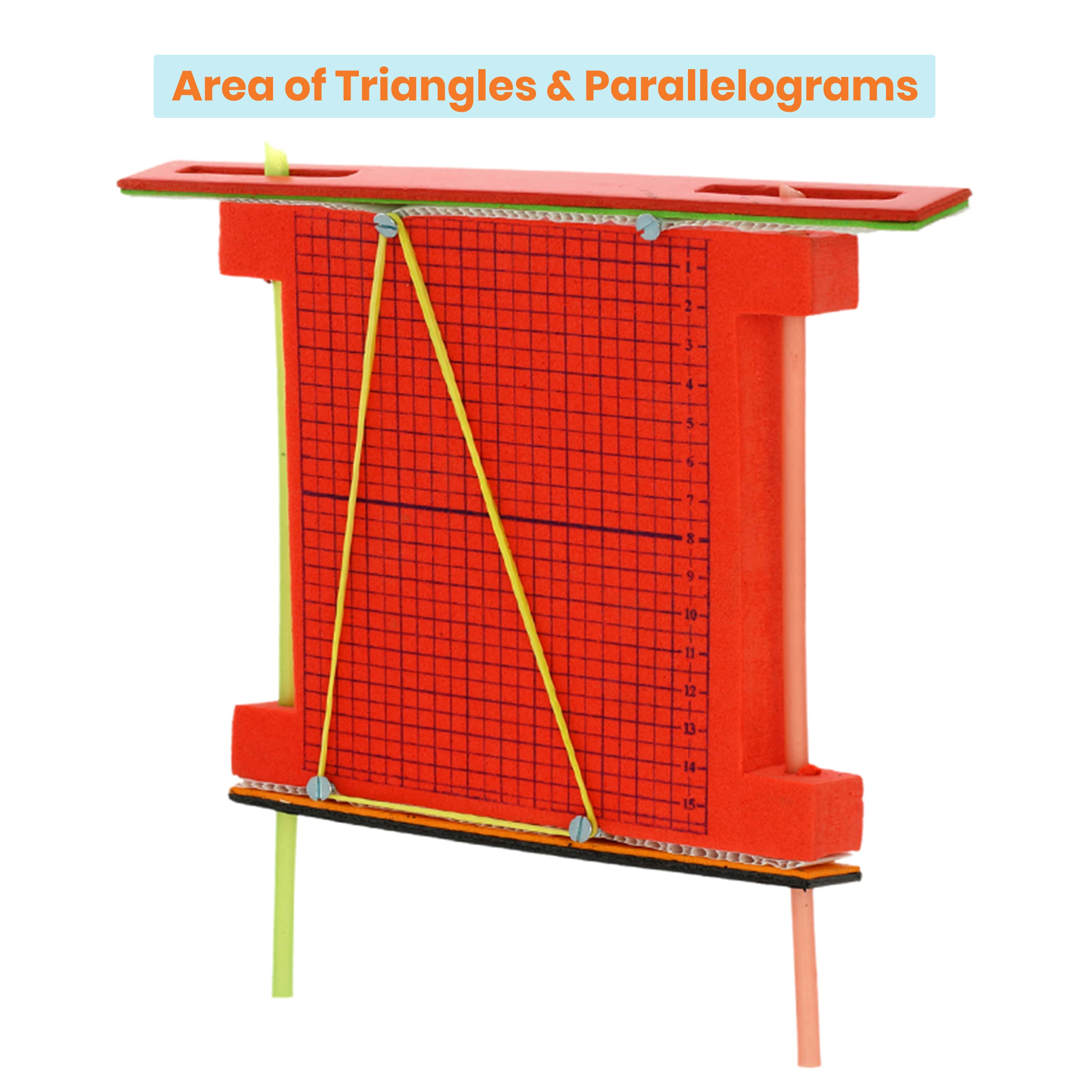### Area of Triangles & Parallelogram tool builder set | Geometry | Math project kit

Rs. 419.00### Activities to teach & practise data representation using Bar graph, Pictograph & Histogram | Math learning activities for kids

Rs. 329.00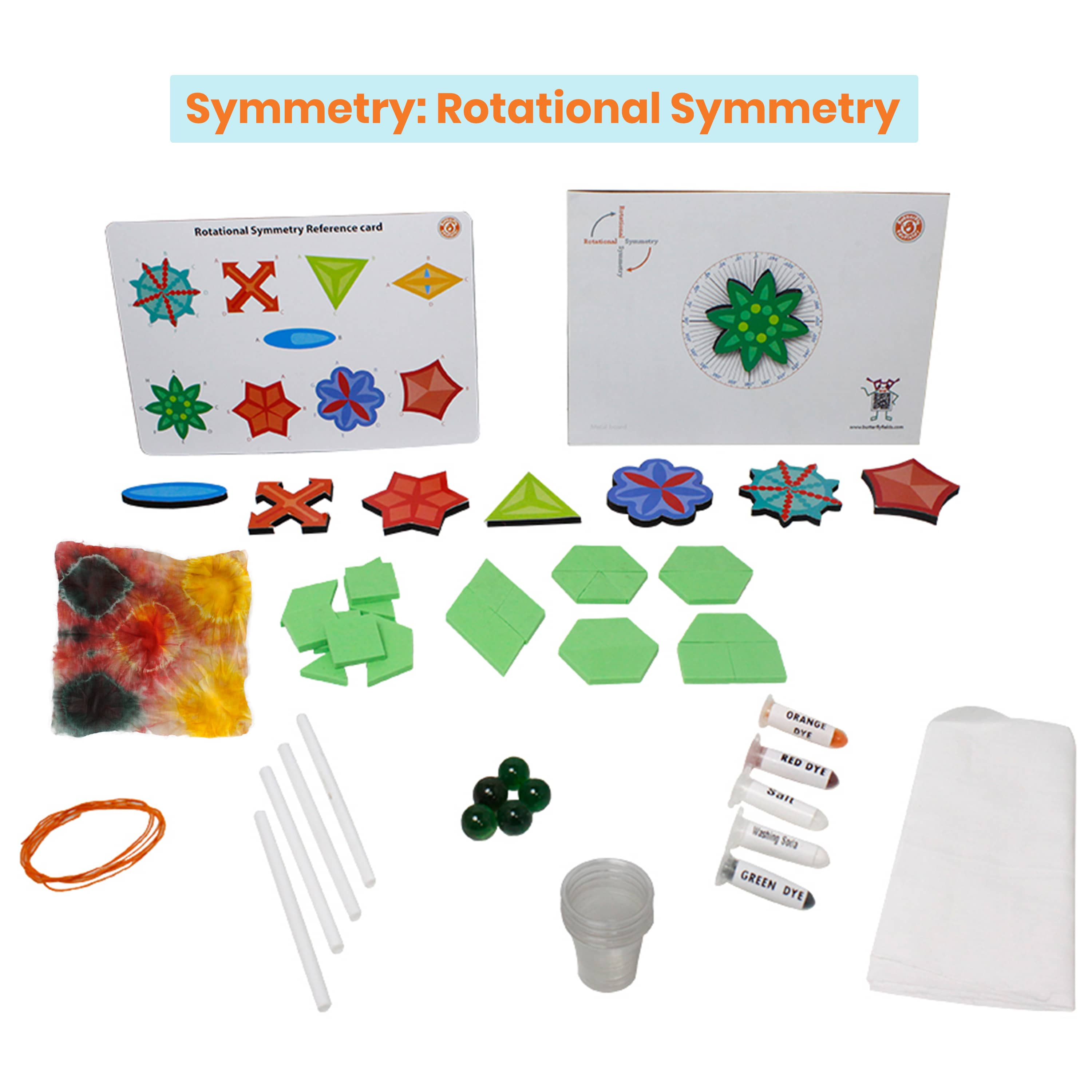### Fun Magnetic shapes set | Line & Rotational symmetry |Math learning kit

Rs. 419.00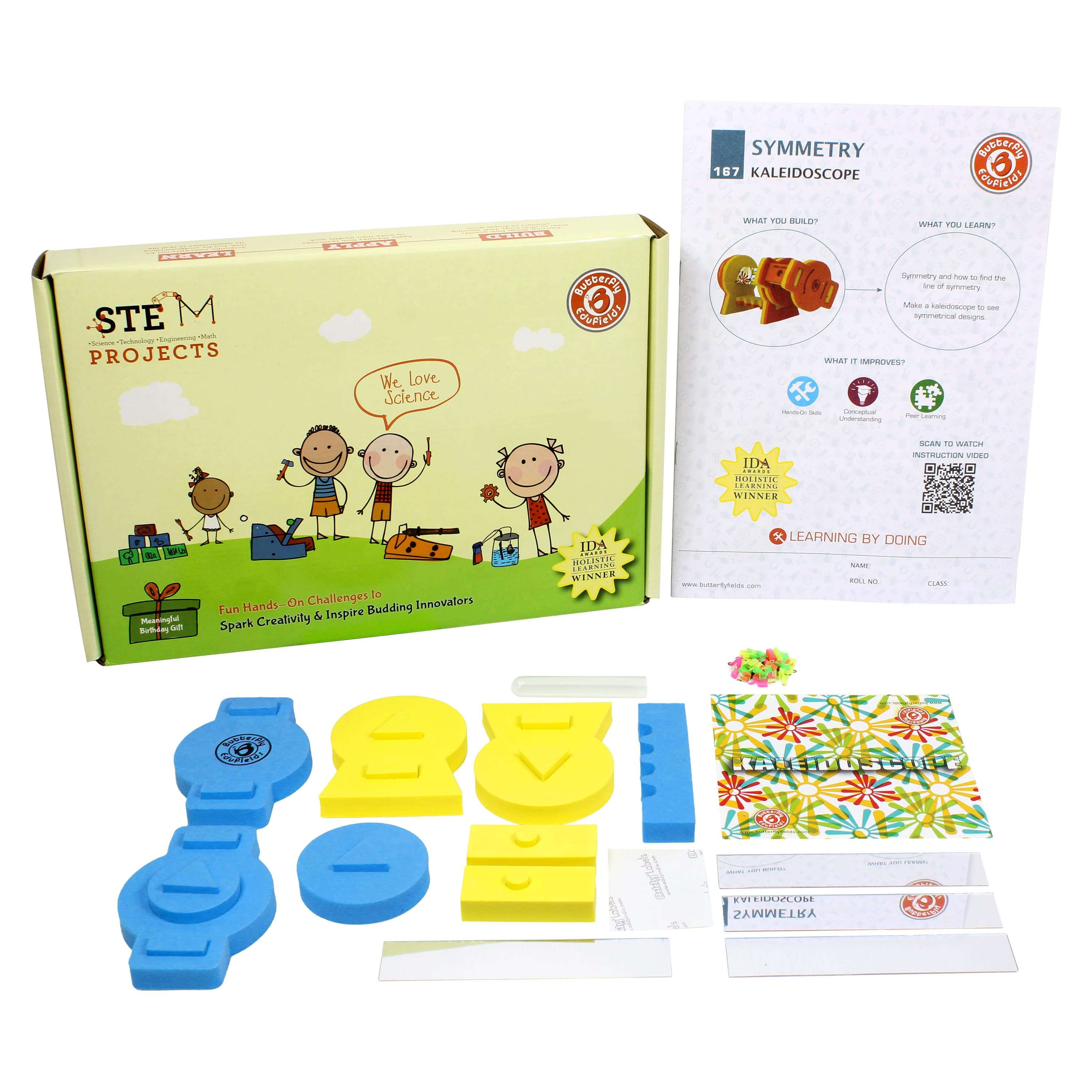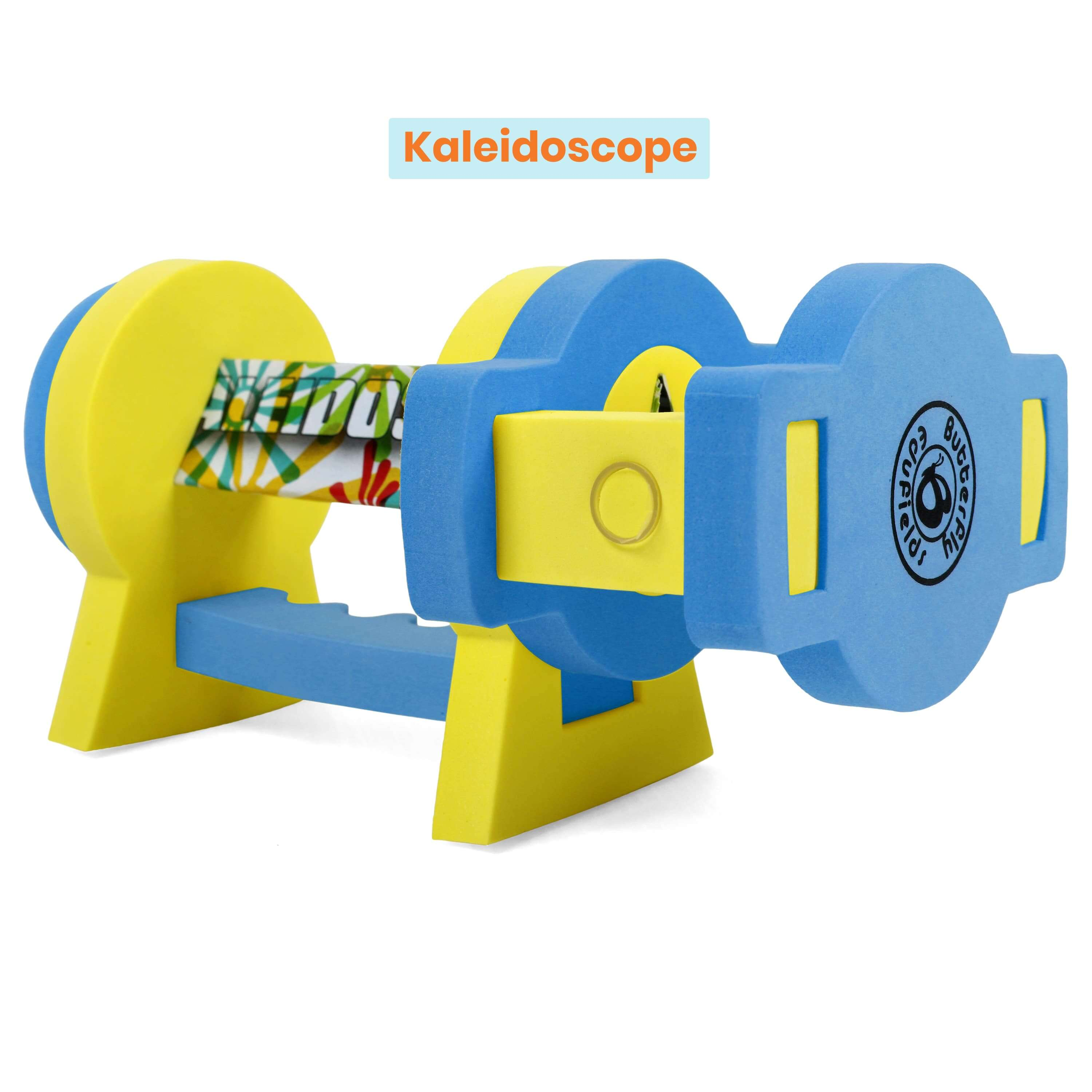### Make your own Kaleidoscope | Reflection,Symmetry | Science project kit

Rs. 449.00
Sunday,Monday,Tuesday,Wednesday,Thursday,Friday,Saturday
January,February,March,April,May,June,July,August,September,October,November,December
Not enough items available. Only [max] left.